## Thursday, February 11, 2016

### How to add jitter to a plot using Python's matplotlib and seaborn

In this blog post, we'll cover how to add jitter to a plot using Python's seaborn and matplotlib visualization libraries. We'll discuss when jitter is useful as well as go through some examples that show different ways of achieving this effect.

## When is adding jitter useful?¶

When graphing a categorical variable vs. a continuous variable, it can be useful to create a scatter plot to visually examine distributions. Together with a box plot, it will allow you to see the distributions of your variables. Unfortunately, if your points occur close together, you will get a very uninformative smear that will look something like the visualization I've generated below:

In :
```import seaborn as sns
import matplotlib.pyplot as plt
%matplotlib inline
import warnings
warnings.filterwarnings('ignore')

sns.set(style="white", color_codes=True)
sns.stripplot(x='species', y='petal_length', data=iris)
sns.despine()
```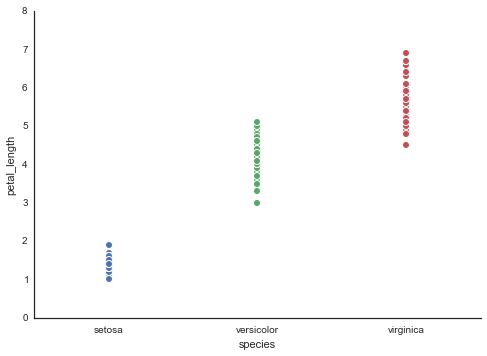Unfortunately, this tells us nothing about the distribution of our variables along the y axis. While we could use a number of other plots, such as a box or violin plot, in certain cases, it can be helpful to use a simple scatter plot. For example, we can have the dots change in colour based on a third variable in order to have a better idea of the relationship between a categorial variable, a continuous variable, and a third variable.

One way of making the scatter plot work is by adding jitter. With the jitter, a random amount is added or subtracted to each of the variables along the categorical axis. Where before, we may have had a categorical value vector that looked something like [1,2,2,2,1,3], post-jitter, they would look something like [1.05, 1.96, 2.05, 2, .97, 2.95]. Each value has had somewhere between [-0.05,0.05] added to it. This then means that when we plot our variables, we'll see a cloud of points that represent our distribution, rather than a long smear:

In :
```sns.stripplot(x='species', y='petal_length', data=iris, jitter=True)
sns.despine()
```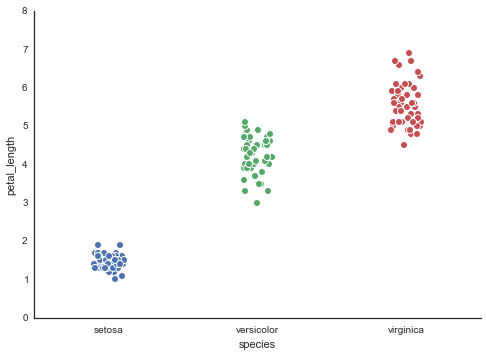We can now see the shape of our data much more easily.

## How do we add jitter using Python's visualization tools?¶

If you're using matplotlib and seaborn, this is fairly straightforward. As you can see in the last cell, we simply set the 'jitter' function to True. You can also set the jitter function to a certain value to give your points more or less jitter -- depending on the data set, you may need to play around with the jitter value to get to a point where you can clearly see the shape of your data.

A few other options are available to you, including removing the points' default white edges to more clearly see the shape of the data:

In :
```sns.stripplot(x='species', y='petal_length', data=iris, jitter=True,
edgecolor='none') # remove the points' default edges
sns.despine()
```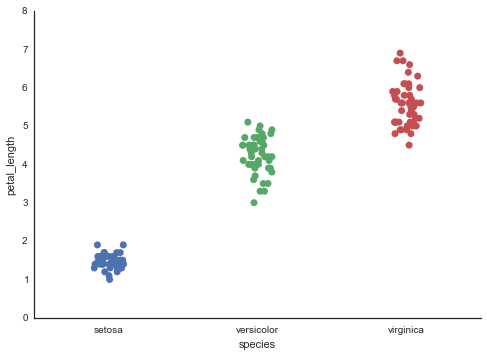Or even making the points somewhat translucent so that the overlap of points is more readily visible.

In :
```sns.stripplot(x='species', y='petal_length', data=iris, jitter=True, edgecolor='none', alpha=.40)
sns.despine()
```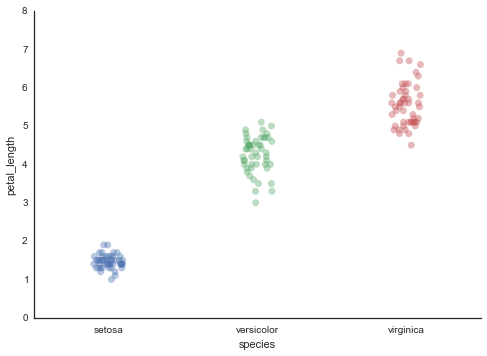This effect can be made more clearly noticeable by increasing the size of the points themselves:

In :
```sns.stripplot(x='species', y='petal_length', data=iris,
size=16, alpha=.2, jitter=True, edgecolor='none')
sns.despine()
```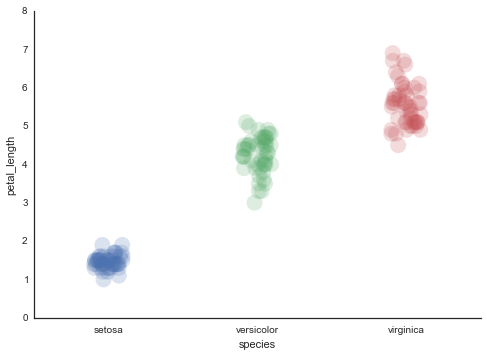Now we can go ahead and easily plot categorical vs. continuous variables using jitters, and changing the translucency, shape, and edge character of the points themselves. Lastly, here's a quick illustration of a jittered scatterplot of a continuous variable vs. 2 other variables:

In :
```import matplotlib.colors as mcolors
import matplotlib.cm as cm

plot = sns.stripplot(x='species', y='petal_length', hue='petal_width', data=iris,
palette='ocean',
jitter=True, edgecolor='none', alpha=.60)
plot.get_legend().set_visible(False)
sns.despine()
iris.describe()

# Drawing the side color bar
normalize = mcolors.Normalize(vmin=iris['petal_width'].min(), vmax=iris['petal_width'].max())
colormap = cm.ocean

for n in iris['petal_width']:
plt.plot(color=colormap(normalize(n)))

scalarmappaple = cm.ScalarMappable(norm=normalize, cmap=colormap)
scalarmappaple.set_array(iris['petal_width'])
plt.colorbar(scalarmappaple)
```
Out:
`<matplotlib.colorbar.Colorbar at 0x111ef62b0>`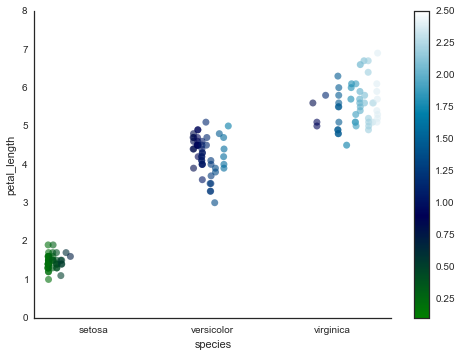As you can see, this graph is rather useful -- we can see that the petal lengths tend to be smaller for type setosa, while type virginica and versicolor tend to have much larger petal types. A quick look at the summary statistics supports the hypotheses we've drawn based on the visualization. You can see them in the table below which groups the dataset by species and then looks at the average value for petal_length and petal_width across each species.

In this case, our scatter plot has allowed us to more clearly explore the relationship between two variables and a third, categorical, variable.

In :
```import numpy as np
grouped = iris[['species', 'petal_length', 'petal_width']].groupby('species')
grouped.aggregate(np.mean)
```
Out:
petal_length petal_width
species
setosa 1.462 0.246
versicolor 4.260 1.326
virginica 5.552 2.026